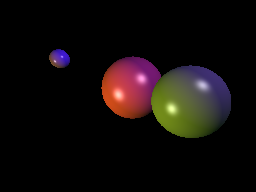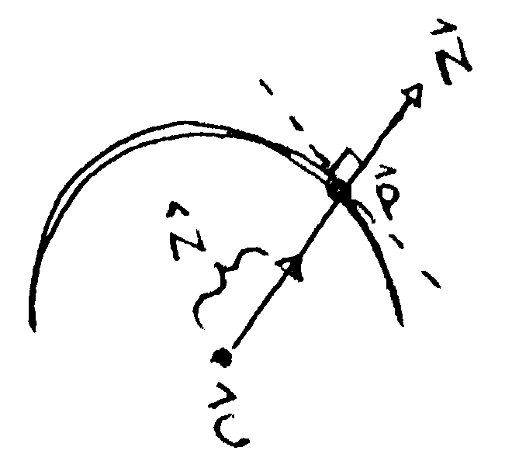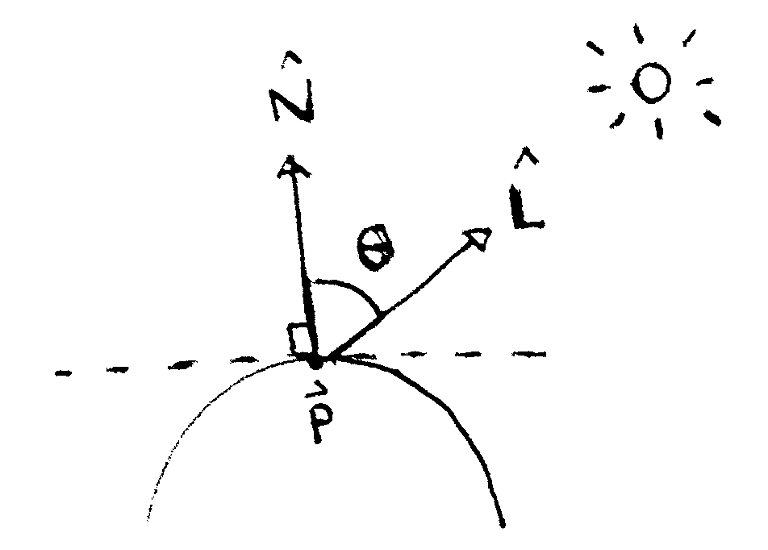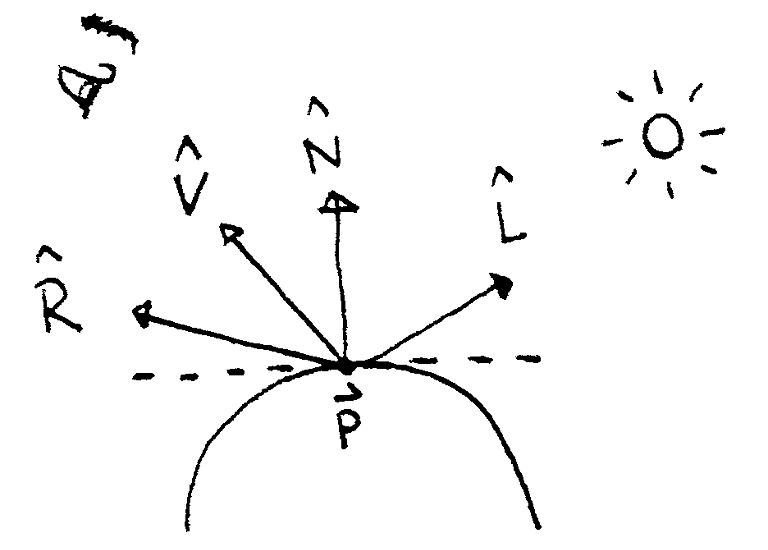# Project 3: Rendering shaded spheres

## Goals

• Represent lights in the scene
• Represent the properties of an object's material
• Implement the Phong illumination model

In this project, we will add lights to the scene and have them interact with the spheres we added before. The spheres themselves will now a have a material that defines how they react to incoming light.Three spheres according to the Phong illumination model.

## Add lights to the world

Create a representation of a light consisting of three pieces of information:

• A location in the world. This is a 3D vector specifying where the light is shining from.
• A diffuse intensity, i_d. This is a color.
• A specular intensity, i_s. This is also a color.

Add a few lights to the scene, with different locations and intensities. Also add another color to the scene: the ambient light intensity i_a.

## Add materials to the spheres

Create a representation of a material with four pieces of information:

• An ambient constant, k_a. This is the percentage of ambient light the material reflects. Technically, this should be its own data type with three components, one for each of the red, green and blue components of the incoming light. However, because we're representing colors with components in the range [0, 1], we can simply reuse the color data type.

• A diffuse constant, k_d. This is the percentage of diffuse light the material reflects.

• A specular constant, k_s. This is the percentage of specular light the material reflects.

• A shininess factor, alpha. This is a scalar. The higher the value, the shinier the material.

Give each sphere a material with the above properties.

## Calculate the point of intersection and surface normal

In the last project, we found certain rays intersected with certain spheres. Each time this happens, do the following:

Calculate the surface normal, hat bbN, on the sphere at the point of intersection, vec bbp. Given the value of t at the intersection, the point of intersection is the ray evaluated at that t: vec bbp = vec bbo + t vec bbd.To find the surface normal hat bbN at point vec bbp, take the vector between vec bbp and the sphere's center vec bbc, then normalize it.

Given the point of intersection, the surface normal on the sphere points in the direction vec bbN = vec bbp - vec bbc, where vec bbc is the center of the sphere. Normalize this vector to get hat bbN.

Do this after you've found the closest intersection, instead of while you're looping through all the objects trying to find the closest one. That way, you won't have to do these calculations every time you find a new candidate for the closest intersection.

## Calculate the ambient term of the Phong illumination model

Previously, you were using the color assigned to the intersected sphere as the color of the pixel corresponding to a ray colliding with that sphere. Now, construct a new color based on the Phong illumination model. Start with the ambient term, k_a i_a.

## Calculate the diffuse term

Iterate through each light in the scene. For each light, calculate the light vector, hat bbL. To calculate this, take the location of the light, subtract the intersection point and normalize the resulting vector.

If the light vector does not point in roughly the same direction as the normal vector, the light is facing the inside of the sphere. This happens when \hat bbN * \hat bbL < 0. Ignore that light in this and the next step.

For each light we are not ignoring, calculate the diffuse component, k_d i_d (\hat bbN * \hat bbL).The diffuse component at a point vec bbp depends on the angle between two vectors. The first vector is the surface normal at the point, hat bbN. The second is the normalized vector between the point and the light, hat bbL.

Add all these terms to the color you're building up for that pixel.

## Calculate the specular term

For that same light, calculate the reflectance vector: hat bbR = 2 (hat bbN * hat bbL) hat bbN - hat bbL. Also, calculate the view vector hat bbV by taking vec bbC - vec bbp, where vec bbC is the position of the camera, and normalizing it. Now calculate the specular component, k_s i_s (hat bbV * hat bbR)^alpha.The specular highlight at a point vec bbp depends on the angle between the reflectance vector hat bbR and the viewing angle hat bbV. The reflectance vector depends on the light vector hat bbL.

Add all these terms to the color you're building up for that pixel.

## Clamp the resulting color

You now have a color consisting of all the contributions from the last three steps. This is the color of the pixel corresponding the ray being considered.

Based on how you've set up your lights and materials, it's possible this color has a component greater than 1. To avoid problems in this case, clamp each component to the range [0, 1]: if a component is below 0, set it to 0, and if it is above 1, set it to 1.

In the reference image, we added three spheres of three different colors. There are also two lights on opposite ends of the spheres. The resulting image looks like this: